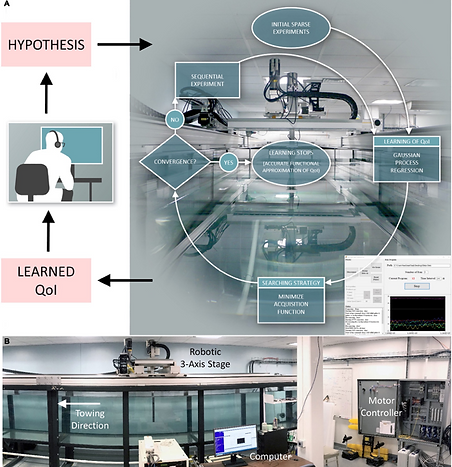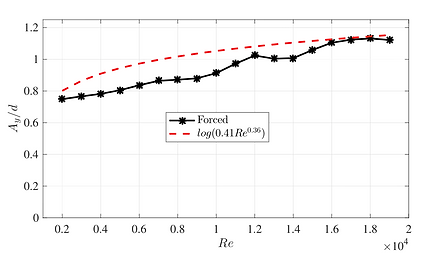top of pageConfession by a "lazy" fluid mechanist

Once, there was a "lazy" fluid mechanist who dreamed of a smart machine that could replace him to perform, analyze and design the experiments to study the fluid-structure dynamics.01. Intelligent Towing Tank (Paper 01, Paper 02, Demonstration Code)Fig. 01, Schematic image of the ITT

I designed and constructed the ITT, shown in Fig. 01.

The process of the ITT is as follows:

• A hypothesis is proposed (such a hypothesis is human generated, or, in the future, may be synthesized in coordination by robots, computers and humans ).

• The ITT performs the adaptive sequential experiment to learn target quantities of interests (QoIs), interrupted only by pause periods between experiments to avoid cross-contamination of the results between successive experiments.

• Upon convergence, the results of learned QoIs are further post-processed to examine the validity of the hypothesis. During sequential experimental testing there is no human-in-the-loop.

The learning algorithm running on the ITT is Gaussian process regression (GPR). The input for the next experiment in sequence is decided by maximizing the standard deviation,  quantified uncertainty by the GPR.

We let the ITT study one of the canonical FSI problems: the hydrodynamic forces of a rigid cylinder undergoing vortex-induced vibrations (VIVs). A very nice demonstration of the phenomenon can be found here by Dr. Gabe Weymouth.

Example 01: A demonstration of ITT learning process on the lift coefficient in phase with the velocity (Clv) of a rigid cylinder only vibrating in the cross-flow direction, shown in Fig. 02. (Publication)Fig. 02, A rigid cylinder forced to vibrating in the uniform inflow

Mov. 01, ITT learning the Clv of CF-only vibrating rigid cylinder.

Example 02: The Reynolds number effect on the CF-only oscillating cylinder. (Preprint)Fig. 03, Comparison between the predicted CF amplitude for a zero-damped cylinder from the forced (current) and free vibrations 

Mov. 02, ITT learning the Reynolds number effect on a CF-only vibrating rigid cylinder between Re = 1,200 to 19,000.

02. Physical-Informed Reinforcement Learning on Flow Control (Ongoing)

03. System Parameter Estimation using PINN (Ongoing)

 J. Wise, These robots are learning to conduct their own science experiments. Bloomberg Businessweek. (April 11, 2018).

 R. N. Govardhan, C. H. K. Williamson, Defining the ‘modified Griffin plot’ in vortex-induced vibration: revealing the effect of Reynolds number using controlled damping. J. Fluid. Mech. 561, 147-180 (2006).

ITT-r
RL-r
PINN
citation 01
bottom of page